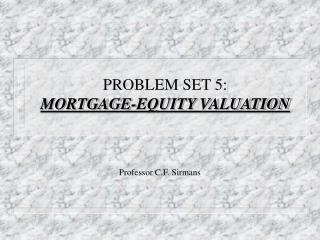DownloadDownload PresentationPROBLEM SET 5: MORTGAGE-EQUITY VALUATION

# PROBLEM SET 5: MORTGAGE-EQUITY VALUATION

Download Presentation## PROBLEM SET 5: MORTGAGE-EQUITY VALUATION

- - - - - - - - - - - - - - - - - - - - - - - - - - - E N D - - - - - - - - - - - - - - - - - - - - - - - - - - -
##### Presentation Transcript

1. PROBLEM SET 5:MORTGAGE-EQUITY VALUATION Professor C.F. Sirmans

2. PROBLEM 1

3. PROBLEM 1

4. PROBLEM 1

5. PROBLEM 1

6. PROBLEM 1

7. PROBLEM 1

8. PROBLEM 1

9. PROBLEM 1

10. PROBLEM 1

11. PROBLEM 1

12. PROBLEM 1

13. PROBLEM 1

14. PROBLEM 2 • PROBLEM: • An apartment investment has the following assumption: • NOI = \$10,000 per year (constant) • N = 10 years • Reversion = Selling price equal to today’s value (no change in property values) • What is the value of this investment?

15. PROBLEM 2

16. PROBLEM 2 • With Debt: • If the project is financed as follows: • 75% loan-to-value • 9% interest rate (interest only) • Balloon payment is 10 years • 12% equity yield

17. PROBLEM 2

18. PROBLEM 3

19. PROBLEM 3

20. PROBLEM 3

21. PROBLEM 3

22. PROBLEM 3

23. PROBLEM 3

24. PROBLEM 3 • COMMENT: The value of this property is derived from the cash flows that is produces. These cash flows come from two sources; 1) operating cash flows, and 2) equity reversion. Before tax operating cash flows are essential to the NOI minus debt service. If NOI and debt service are both constant, then they may be treated as an annuity. Before tax equity reversion must take into account selling expenses, growth rates, and the unpaid mortgage balance. It is important to note that zero taxes are considered in this problem. One might think that zero taxes are not realistic, however, there are several instances, such as non-profit organizations or pension funds, when this is in fact the case.

25. PROBLEM 4

26. PROBLEM 4

27. PROBLEM 4

28. PROBLEM 4

29. PROBLEM 4

30. PROBLEM 5

31. PROBLEM 5

32. PROBLEM 5

33. PROBLEM 5

34. PROBLEM 5